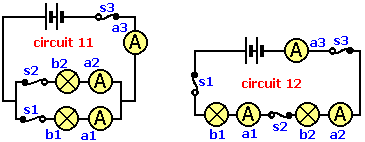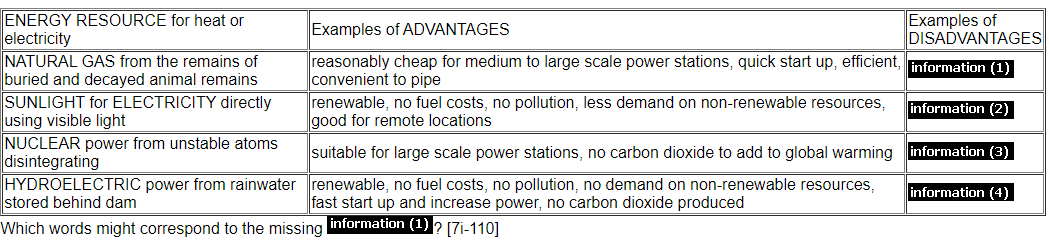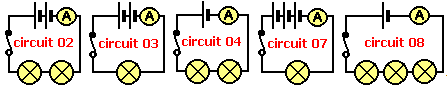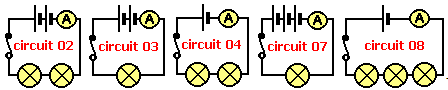# Combined quiz on energy electricity forces and the solar system

#### Quiz Description

In this quiz, we will be looking at energy, electricity, forces and the solar system combined. Practically, you will be amazed to know that solar energy from the sun is used to charge up solar panels and these solar panels generate electricity as a product, which is electrical energy. This is a practical use of energy, and energy conversion makes this possible. On taking this quiz, you will learn things like forms of energy and energy conversion.

Energy is simply the ability or the capacity to do work. It exists in many different forms, electrical, heat or thermal, chemical, light, sound, and mechanical energy. Each of these energy forms can be converted from one to another as by the first law of energy.

Electricity is the flow of charge, and it is a secondary energy source. There are two types of electricity, Static electricity, and current electricity. Static has to do with charges at rest and current electricity studies charges in motion.

Now, let’s talk about forces. A force is that which can set an object in motion, change the direction of motion of an object, and can also stop an object from moving. A good example is an electrical force which is the pure attractive or repulsive force between two charged bodies.

The solar system is made up of our star, the Sun, and everything bound to it by gravity like our planet Earth and all the other planets.

These quizzes will help prepare you for your upcoming exams, be it SATs, GCE, or GCSE. And this particular quiz is a combination of the individual quizzes energy, and electricity as well as the solar system quizzes. More physics quizzes are also available. Take the quiz and see how ready you are for any upcoming test/exam.
Best of Luck!

Which matches the length of night-time on the equator?

Correct
• year
• day
• month
• half a day

Which explains why Mars has 'seasons'?

Correct
•   the spin axis is tilted
•  the same side always faces the Sun
•   it moves nearer to, or further from, the Sun
•  it rotates around a spin axis

Which planet is the 4th in order from the Sun?

Correct
• Saturn
• Venus
• Mars
• Earth

Which of the following planets has the shortest year?

Correct
• Mercury
• Pluto
• Venus
• Saturn

Which statement about electricity and circuits is TRUE?

Correct
•  more bulbs wired in series decreases the total resistance
•  the circuit does not have to be complete for a current to flow
•  to be effective cells must be connected + to -
•  the conventional current flows from - to -

Which of the following is a 'force'?

Correct
• light
• kinetic
• friction
• electricity

Calculate the block volume if L = 3 cm, B = 6 cm and H = 2 cm

Correct
• 54 cm3
•  18 cm3
• 15 cm3
• 36 cm3

Solar __(1)__ can convert __(2)__ directly into __(3)__ but they are __(4)__. Dark solar panels fitted in a roof, readily __(5)__ radiation to heat water. A curved __(6)__ can also be used to collect and concentrate the heat radiation to cook food on a barbecue!  Which word is missing from __(3)__?

Correct
• absorb
• costly
• sunlight
• electricity

We measure __(1)__ using a __(2)__. One __(3)__ of temperature is called the degree __(4)__ (oC). Temperature is a measure of how __(5)__ or cold something is and, because it can change, it is known as a __(6)__.  Which word is missing from __(5)__?

Correct
• hot
• variable
• thermometer
• unit

Which is a renewable source of energy?

Correct
• battery
• peat
•  wind generator
• oil

Which is the path of an object moving around another in space?

Correct
• planet
• orbit
• constellation
• asteroid

Which of the following is TRUE when comparing the two circuits 11 and 12? Note that the bulbs are identical and the batteries identical.Correct
•  if switch s2 is opened in both circuits, all the bulbs go out in circuit 11 and only bulb b1 in circuit 12
•  if ammeter a3 reads 0.4A in both circuits, ammeter a2 reads 0.2A in circuit 11 and ammeter a2 reads 0.4A in circuit 12
•  if ammeter a3 reads 0.4A in both circuits, ammeter a1 reads 0.4A in circuit 11 and ammeter a1 reads 0.2A in circuit 12
•  if ammeter a3 reads 0.4A in both circuits, ammeter a2 reads 0.4A in circuit 11 and ammeter a2 reads 0.2A in circuit 12

Analyse the information belowCorrect
•  unreliable, no good for large scale power
•  very costly to build, disruption or removal of wildlife habitat or farmland, limited suitable valley locations
•  medium pollution, carbon dioxide adds to global warming, non-renewable (90 years left?)
•  toxic radioactive waste, accidents potentially very serious, very costly to build and dismantle, non-renewable

The five circuits show various arrangements of identical cells and identical batteries. If the ammeter reading in circuit 02 is 0.3A, in which circuit will the ammeter reading be 0.6A?Correct
•  circuit 08
•   circuit 03
•  circuit 07
•  circuit 04

The five circuits show various arrangements of identical cells and identical batteries. If the ammeter reading in circuit 02 is 0.3A, in which circuit will the ammeter reading be 0.15A?Correct
•  circuit 08
•  circuit 03
•   circuit 07
•  circuit 04